# AP Chemistry Practice Test 41

### Test Information12 questions18 minutes

1. A chemist has a 500 mL beaker with a small amount of mercury(II) oxide, HgO, on the bottom. Mercury(II) is insoluble in water but will dissolve in strong acid. She adds 250 mL of water and begins adding 1.0 M hydrochloric acid. She continues adding acid until the solid just dissolves. She then tests the solution and finds that it is nonconducting. Which of the following best represents the result for the solution just as the last of the solid dissolves?

2. A dilute aqueous solution of potassium bromide, KBr, is heated to the boiling point. Which of the following best represents this system?

3. The average distribution of bromine isotopes on Earth is in the following table: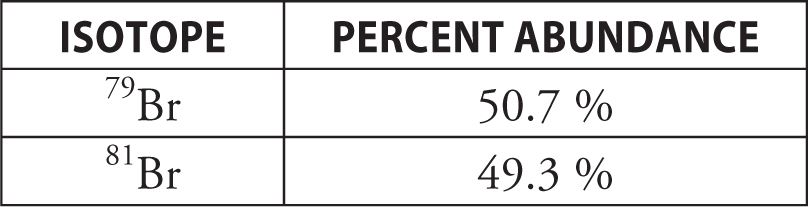The highest mass peaks in the mass spectrum of methyl bromide, CH3Br, are at 94 and 96 atomic mass units (there are smaller peaks corresponding to the small quantities of the less common isotopes of carbon and hydrogen). The spectrum plot below is of this region.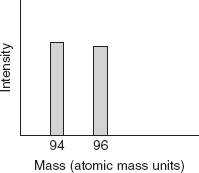The mass spectrum for methylene bromide, CH2Br2, is more complicated in that there are three main peaks in the highest mass region (again ignoring minor contributions from other carbon and hydrogen isotopes). Methylene bromide has a mass of about 174 atomic mass units.

Which of the following is the best representation of the mass spectrum of CH2Br2 in the 174 region?

4. There are three steps in the formation of a solution. It is necessary to overcome the intermolecular forces present within the solute. It is also necessary to overcome the intermolecular forces present within the solvent. Both of these steps require energy related to the strength of the intermolecular forces. The final step in the formation of a solution involves the creation of new intermolecular forces between the solute and solvent. This energy release is related to the strength of the intermolecular forces created. Which of the following illustrates a situation most likely to require the least amount of energy to overcome the intermolecular forces?

5.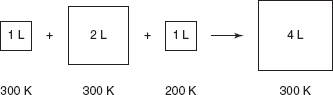The contents in the three containers on the left in the diagram above are transferred to the container on the right. The volumes of the original containers are exactly the values indicated. The pressure in the first three containers is 1.0 atm. What is the pressure in the container on the right?

6.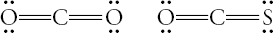The diagram above shows the structure of molecules of CS2 and COS. The boiling point of COS is 223 K, and the boiling point of CS2 is 319 K. Which of the following is the best explanation of why the boiling point of CS2 is higher?

7.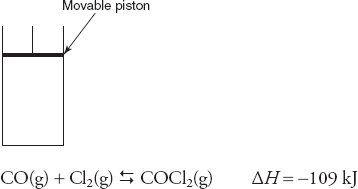The above equilibrium is established in a closed system with a movable piston. After establishing equilibrium, the piston is rapidly moved up (pressure change). Which of the following graphs best illustrates the rate of the reverse reaction as the system returns to equilibrium?

8. The proposed mechanism for the above reaction is as follows: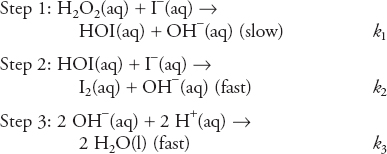What is the rate law expression for this reaction?

9. A 30.00 g sample of metal (X) was heated to 100.00°C. This sample was clamped in contact with a 40.00 g sample of a different metal (Y) originally at 25.00°C. The final temperature of the two metals was 37.30°C and no heat was lost to the surroundings. What is one possible conclusion from this experiment?

10.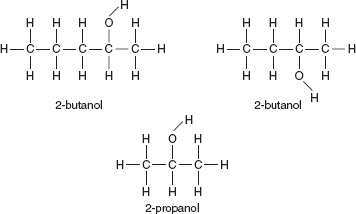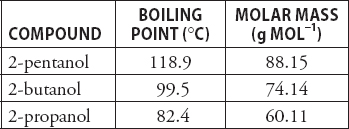Which of the following best explains why the boiling point of 2-propanol is lower than the other two compounds in the diagram and table above?

11.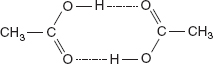The Dumas method is a procedure for determining the molar mass of a gas. In this procedure the mass of a gas is divided by the moles of gas determined from the ideal gas equation (n = PV/RT). The molar masses of some compounds, such as acetic acid, illustrated above, show significant deviations from the “correct” values. Why does the presence of dimers as illustrated make it unlikely to obtain an accurate molar mass of acids, such as acetic acid?

12. In which of the following groups are the species listed correctly in order of increasing ionization energy?